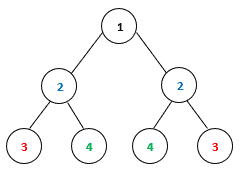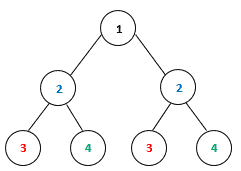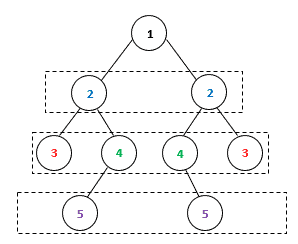## 1. Introduction

In Computer Science, a binary tree is a data structure in which each node has at most two children.

In this tutorial, we’ll show how to check if a binary tree is symmetric.

## 2. Symmetric Binary Tree

In a binary tree, each node has two subtrees, left subtree and right subtree. A subtree can be empty, a single node, or another binary tree. A binary tree is symmetric if the root node’s left subtree is a mirror reflection of the right subtree. For example, the following binary tree is symmetric:The following binary tree is not symmetric, although the two subtrees have the same tree structure:## 3. Recursive Solution

Based on the symmetric definition, we can use the following rules to check whether two binary trees are a mirror reflection of each other:

• The two root nodes have the same value
• The left subtree of one root node is a mirror reflection of the right subtree of the other root node

It is easy to transform these conditions into a recursive algorithm:In this algorithm, we use a recursive function,, to check whether two binary trees are a mirror reflection of each other. Firstly, we do some sanity checks to handle cases where at least one of the input binary trees is empty. Then, we apply the symmetric rules on the two non-empty trees by recursively calling thefunction.

At the high level, we can call thefunction on our input binary tree’s left subtree and right subtree. Since we traverse the entire input tree once, the running time of this algorithm is, whereis the total number of nodes in the tree.

## 4. Iterative Solution

We can also solve this problem in an iterative way by traversing the binary tree level by level. At each level, the tree nodes should be symmetric:We can implement this tree traversal algorithm with the help of a queue data structure:In this iterative algorithm, we first construct a queue that contains the two children of the root node. Then, in each iteration of the loop, we extract two nodes from the queue and compare their values. Also, the right and left children of the two nodes are inserted in the queue in the opposite order for future symmetric detections. We continue this process until we traverse all tree nodes or we detect that the tree is not symmetric.

Same as the recursive solution, we also traverse the entire input tree once. Therefore, the running time of the iterative algorithm is, whereis the total number of nodes in the tree.

## 5. Conclusion

In this tutorial, we showed some examples of symmetric binary trees. Also, we discussed two algorithms that can detect whether a binary tree is symmetric in linear time.